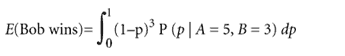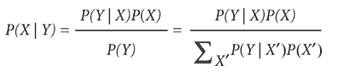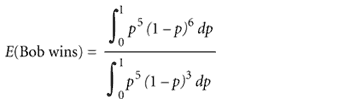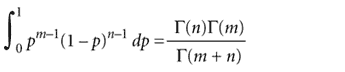Thank you for visiting nature.com. You are using a browser version with limited support for CSS. To obtain the best experience, we recommend you use a more up to date browser (or turn off compatibility mode in Internet Explorer). In the meantime, to ensure continued support, we are displaying the site without styles and JavaScript.

# What is Bayesian statistics?

There seem to be a lot of computational biology papers with 'Bayesian' in their titles these days. What's distinctive about 'Bayesian' methods?

There are excellent introductory books on Bayesian analysis1,2,3, but the key ideas behind the buzzword can be grasped quickly. Consider the following gambling puzzle—one that has ancient roots in the origins of both classical and Bayesian probability theory.

The table game

Alice and Bob are playing a game in which the first person to get 6 points wins. The way each point is decided is a little strange. The Casino has a pool table that Alice and Bob can't see. Before the game begins, the Casino rolls an initial ball onto the table, which comes to rest at a completely random position, which the Casino marks. Then, each point is decided by the Casino rolling another ball onto the table randomly. If it comes to rest to the left of the initial mark, Alice wins the point; to the right of the mark, Bob wins the point. The Casino reveals nothing to Alice and Bob except who won each point.

Clearly, the probability that Alice wins a point is the fraction of the table to the left of the mark—call this probability p; and Bob's probability of winning a point is 1 − p. Because the Casino rolled the initial ball to a random position, before any points were decided every value of p was equally probable. The mark is only set once per game, so p is the same for every point.

Imagine Alice is already winning 5 points to 3, and now she bets Bob that she's going to win. What are fair betting odds for Alice to offer Bob? That is, what is the expected probability that Alice will win?

If p were known, this would be easy

Because Alice just needs one more point to win, Bob only wins the game if he takes the next three points in a row. The probability of this is (1 − p)3; Alice will win on any other outcome, so the probability of her winning is [1 − (1 − p)3]. If Alice knew p, it would be easy for her to calculate fair odds. For instance, if the mark were exactly in the middle of the table (or if this were the 'coin game,' where points are decided by flipping a fair coin), p would be 0.5; the probability that Bob would win would be (1 − 0.5)3, or 1/8; and the probability that Alice would win would be 7/8; fair odds would be 7:1.

What we're doing here is calculating the probability of observing some data (the outcomes of up to the next three points) given a probability model (the probability p). The general notation for such a probability is P(data | model), where the '|' sign means 'given' or 'conditional upon.'

Calculating the probability of an observed outcome given known parameters and known hypotheses tends to be a familiar process, especially if we're talking about outcomes of flipping coins, rolling dice or drawing white and black balls from urns. Interestingly, though, the 'share problem' (A leads B 5:3 in a coin-flipping game to 6; the game is interrupted; how to fairly split the pot?) was controversial for centuries after it was first proposed in the 1300s. Published solutions included 2:1 and 3:1 odds, and one mathematician sniffed at another's solution, “there is an evident error in the determination of the shares that even a child should recognize”—but gave no answer himself4. (Statistics has changed since the Renaissance; peer review is much the same.) Blaise Pascal's mid-1600s correspondence with Fermat describing his reasoning in deriving a correct 7:1 solution is considered to be one of the origins of probability theory.

Inferring p from the data

The problem is that Alice and Bob don't know p. The very fact that Alice is ahead 5-3 is evidence that the unknown position of the mark is probably giving Alice an advantage, but the numbers are small, and she can't be sure. Maybe the mark is in Bob's favor and he's just been unlucky so far.

This sets up a scientific inference problem in microcosm. We have a limited amount of data: Alice is winning 5-3. We are interested in inferring an unknown 'hypothesis': the value of p. We want to use this inference to predict future events: how probable is it that Alice will win?

One approach would be to make a maximum likelihood estimate of the unknown parameter p. This is the frequency at which Alice has won so far, 5/8. From this, we estimate that Bob's probability of winning is (3/8)3 = 27/512, and Alice's probability of winning is 485/512; fair odds would be about 18:1. But, as we will see, this is way off.

The Bayesian solution

The Bayesian approach is to write down exactly the probability we want to infer, in terms only of the data we know, and directly solve the resulting equation — which forces us to deal explicitly with all mathematical difficulties, additional assumptions and uncertainties that may arise. One distinctive feature of a Bayesian approach is that if we need to invoke uncertain parameters in the problem, we do not attempt to make point estimates of these parameters; instead, we deal with uncertainty more rigorously, by integrating over all possible values that a parameter might assume.

Here, for instance, what we want to know is the expected probability that Bob will win (call it E). By definition, this is the weighted average of (1 − p)3 over all possible values of p:where the (1 − p)3 term is the probability that Bob wins given a particular choice of p and the P(p | A=5, B=3) term is the probability that that particular choice of p is the correct one, given the observed data that the score is Alice 5, Bob 3.

What is P(p | A=5, B=3)? The probability of the parameter p given the data is not the same thing as the more familiar calculation of P(A=5, B=3 | p), the probability of the data given a known parameter p. It is a so-called inverse probability problem. Rather than P(data | model), we need P(model | data).

The solution to inverse probability problems is the grandiosely named “Bayes' theorem”, which actually is a trivial algebraic truism for two random variables X and Y:or, in this case,That is, the probability of a particular choice of p given the data (the 'posterior probability' of p) is proportional to the probability that we would get the observed data if that p were true (the 'likelihood' of p) multiplied by the a priori probability of this p relative to all other possible values of p (the 'prior probability' of p). To make this come out as a probability, we divide by a summation over all possible values of p; because p is a continuous variable, this means an integration from p = 0 to p = 1. The use of inverse probability calculations and Bayes' theorem is a second distinctive feature of Bayesian approaches.

The likelihood term is the term we know how to calculate; P(A=5,B=3 | p) is a binomial (8!/5!3!)p5(1 − p)3. The prior term P(p) is potentially problematic. By definition, P(p) is a probability of p before any data have been observed. How do we know anything about p before we've seen any data?

A crucial feature of the 'table game' is that P(p) is well-defined: the game is contrived such that p is picked from a uniform distribution. Because it's uniform, it's a constant, and it cancels out of the Bayes equation; after some algebraic rearrangement, we're left with:It happens that these integrals have analytic solutions. A 'beta integral' iswhere Γ(x) is a gamma function, a generalization of the better-known factorial function to real numbers: Γ(n + 1) = n! for an integer n. So, plugging in and solving, we get an answer of (5!6!/12!)/(5!3!/9!) = 1/11 for Bob's expected probability of winning, and Alice's expected probability is 10/11. Thus, the Bayesian calculation estimates fair odds to be 10:1—which is verifiably correct, as we'll see below.

Difficulties with Bayesian statistics

Bayesian analysis (explicit probabilistic inference) is an attractively direct, formal means of dealing with uncertainty in scientific inference, but there are three important difficulties.

One difficulty is computational. Bayesian calculations almost invariably require integrations over uncertain parameters. These integrations often have no analytical solution, and instead require computationally intensive numerical integration (such as Markov-chain Monte Carlo methods). Until the advent of computers, Bayesian approaches often weren't feasible.

Second, Bayesian methods require specifying prior probability distributions, which are often themselves unknown. Bayesian analyses generally assume so-called 'uninformative' (often uniform) priors in such cases. Introducing subjective assumptions into an inference is unpalatable to some statisticians. The usual counterargument is that non-Bayesian methods make comparable assumptions implicitly, and it's probably better to have one's assumptions out in the open.

Third, though Bayes' theorem is trivially true for random variables X and Y, it is not clear to everyone that parameters or hypotheses should be treated as random variables. Everyone accepts that we can talk about the probability of observed data given a model, where we mean the frequency with which we would obtain those data in the limit of infinite trials. But if we talk about the 'probability' of a one-time, nonrepeatable event that is either true or false, there is no frequency interpretation, and we are using probability in the sense of a confidence or a degree of belief. This seems common sense, but it remains controversial amongst good statisticians. Using probability to represent a degree of belief is a third distinctive feature of Bayesian approaches.

My 'table game' is adapted from the key example in the landmark, posthumous 1763 paper by the Reverend Thomas Bayes. The beauty of Bayes' table-and-balls analogy is that it circumvented all three difficulties in one stroke, making it possible to think clearly about a verifiable inverse probability problem. Bayes' example provided a physical mechanism for drawing a probability from a uniform prior; the resulting integrals have analytic solutions; and every term has a frequentist interpretation, because we can repeat the physical process of rolling a trial ball to choose p. Indeed, it is easy to verify that the correct answer to the table game problem is 10:1—write a computer program to simulate the table game many times, and count the frequency with which Alice versus Bob ends up winning after a match reaches a 5-3 score in Alice's favor.

Applications in computational biology

There is no shortage of problems in biology where we want to infer something from observed data, but the inference depends on uncertain parameters or missing data in a probability model. For example, in phylogenetic analysis, the probability of an evolutionary tree given some observed DNA sequences is conditional on a multiple alignment, an evolutionary model, and branch lengths on the tree, all of which are subject to substantial uncertainty, but for which traditional methods try to make single point estimates. Using Bayesian methods, we can instead integrate over varying degrees of uncertainty in different aspects of the analysis. The robustness of Bayesian methods in the face of partial information and poorly determined parameters lets us use more complicated, more realistic probability models. This is proving to be highly useful in the 'post-genomic' world of analyzing large, noisy biological data sets.

## References

1. Gelman, A., Carlin, J.B., Stern, H.S. & Rubin, D.B. Bayesian Data Analysis (Chapman & Hall/CRC, Boca Raton, Florida, USA, 1995).

2. MacKay, D.J.C. Information Theory, Inference, and Learning Algorithms (Cambridge Univ. Press, Cambridge, UK, 2003).

3. Jaynes, E.T. Probability Theory: The Logic of Science (Cambridge Univ. Press, Cambridge, UK, 2003).

4. Hacking, I. The Emergence of Probability (Cambridge Univ. Press, Cambridge, UK, 1975).

Authors

## Rights and permissions

Reprints and Permissions

Eddy, S. What is Bayesian statistics?. Nat Biotechnol 22, 1177–1178 (2004). https://doi.org/10.1038/nbt0904-1177

• Issue Date:

• DOI: https://doi.org/10.1038/nbt0904-1177

• ### Interspecific variation in the timing and magnitude of hydraulic redistribution in a forest with distinct water sources

• Michael Belovitch
• Steven Brantley
• Doug P. Aubrey

Plant and Soil (2022)

• ### Dissecting a Criminal Investigation

• D. Kim Rossmo

Journal of Police and Criminal Psychology (2021)

• ### Assessing Bayesian Racism Scale: Measuring Endorsement of Racial Stereotypes

• Stacey Diane Arañez Litam
• Richard S. Balkin

International Journal for the Advancement of Counselling (2021)

• ### A single shot coherent Ising machine based on a network of injection-locked multicore fiber lasers

• Masoud Babaeian
• Dan T. Nguyen
• N. Peyghambarian

Nature Communications (2019)

• ### Nonlinear optical components for all-optical probabilistic graphical model

• Masoud Babaeian
• Pierre-A. Blanche
• N. Peyghambarian

Nature Communications (2018)

## Search